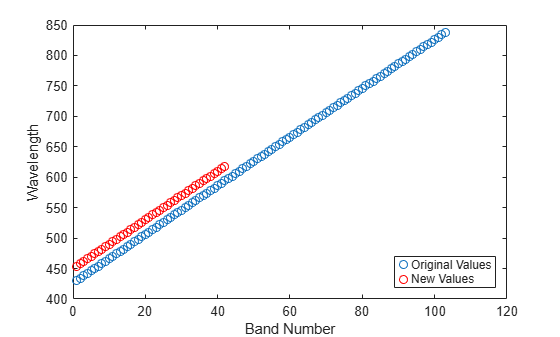# removeBands

Remove spectral bands from data cube

## Syntax

``newhcube = removeBands(hcube,'Wavelength',wlrange)``
``newhcube = removeBands(hcube,'BandNumber',band)``

## Description

example

````newhcube = removeBands(hcube,'Wavelength',wlrange)` removes spectral bands from the data cube within the specified wavelength range. The function returns a new `hypercube` object with the remaining wavelengths, their metadata information, and the corresponding spectral bands from the original data cube.```

example

````newhcube = removeBands(hcube,'BandNumber',band)` removes the spectral bands with the specified spectral band numbers from the hyperspectral data cube. NoteThis function requires the Image Processing Toolbox™ Hyperspectral Imaging Library. You can install the Image Processing Toolbox Hyperspectral Imaging Library from Add-On Explorer. For more information about installing add-ons, see Get and Manage Add-Ons. ```

## Examples

collapse all

Read hyperspectral data into the workspace.

`hcube = hypercube('paviaU.dat');`

Inspect the properties of the `hypercube` object.

`hcube`
```hcube = hypercube with properties: DataCube: [610×340×103 double] Wavelength: [103×1 double] Metadata: [1×1 struct] ```

Find the spectral wavelength range of the hyperspectral data cube.

`range = [min(hcube.Wavelength) max(hcube.Wavelength)]`
```range = 1×2 430 838 ```

Specify the ranges of wavelengths to be remove from the hyperspectral data cube.

`wlrange = [410 450; 620 850];`

Remove the spectral bands that lie in the specified wavelength ranges. The function returns a new `hypercube` object without the removed bands.

`newhcube = removeBands(hcube,'Wavelength',wlrange)`
```newhcube = hypercube with properties: DataCube: [610×340×42 double] Wavelength: [42×1 double] Metadata: [1×1 struct] ```

Plot the original and the new wavelength values.

```figure plot(hcube.Wavelength,'o') hold on plot(newhcube.Wavelength,'or') xlabel('Band Number') ylabel('Wavelength') legend('Original Values','New Values','Location','SouthEast')```Read a hyperspectral data into the workspace.

`hcube = hypercube('paviaU.dat')`
```hcube = hypercube with properties: DataCube: [610×340×103 double] Wavelength: [103×1 double] Metadata: [1×1 struct] ```

Compute five spectrally distinct endmembers of the hyperspectral data cube by using the `ppi` function.

`endmembers = fippi(hcube,5);`

Determine the 10 most informative bands of the input data cube based on the endmembers spectra.

`[~,informativeband] = selectBands(hcube,endmembers,'NumberOfBands',10);`

Find the band numbers of the noninformative bands of the data cube by using the band numbers of the informative bands.

`band = setdiff(1:size(hcube.DataCube,3),informativeband);`

Remove the noninformative bands from the hyperspectral data cube. The function returns a new `hypercube` object with only the most informative bands.

`newhcube = removeBands(hcube,'BandNumber',band)`
```newhcube = hypercube with properties: DataCube: [610×340×10 double] Wavelength: [10×1 double] Metadata: [1×1 struct] ```

Plot the original and the new wavelength values.

```figure plot(hcube.Wavelength,'o') hold on plot(newhcube.Wavelength,'or') xlabel('Band Number') ylabel('Wavelength') legend('Original Values','New Values','Location','SouthEast')```## Input Arguments

collapse all

Input hyperspectral data, specified as a `hypercube` object. The `DataCube` property of the `hypercube` object stores the hyperspectral data cube.

Wavelength range to remove, specified as a K-by-2 matrix. K is the number of wavelength ranges to remove from the input data. Each row is of form [Wmin Wmax]. Wmin and Wmax are the minimum and the maximum wavelengths of the ranges to remove. At least one specified wavelength range must overlap the wavelength value of at least one spectral band within the input `hypercube` object.

Data Types: `single` | `double` | `int8` | `int16` | `int32` | `int64` | `uint8` | `uint16` | `uint32` | `uint64`

Spectral band number to remove, specified as a positive integer or vector of positive integers. All of the specified band numbers must be less than or equal to the number of spectral bands in the input hyperspectral data.

Data Types: `single` | `double` | `int8` | `int16` | `int32` | `int64` | `uint8` | `uint16` | `uint32` | `uint64`

## Output Arguments

collapse all

Output hyperspectral data, returned as a `hypercube` object.